Function Repository Resource:

# PairMap

Map a function to pairs formed from a list and another function

Contributed by: Seth J. Chandler
 ResourceFunction["PairMap"][f,args] creates a data structure whose head is the same as the head of args and each element of which is a list with the first element in args, and the second element has function f applied to that first element. ResourceFunction["PairMap"][f,args,g] applies g to each element in the result. ResourceFunction["PairMap"][f,args,g,h] wraps h around the overall result. ResourceFunction["PairMap"][f] is an operator form of ResourceFunction["PairMap"] that can be applied to arguments.

## Details and Options

ResourceFunction["PairMap"][f,args,g,h] wraps the function h around the output from ResourceFunction["PairMap"][f,args,h].

## Examples

### Basic Examples (3)

Create a list of lists with elements of the form {x,f[x]}:

 In:=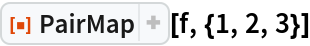Out=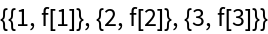Create a list of rules of the form xf[x]:

 In:=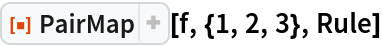Out=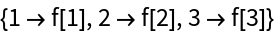Apply an operator form of PairMap to some data:

 In:=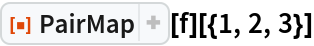Out=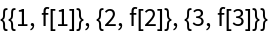### Scope (2)

The head of the result returned by PairMap is the same as the head of args:

 In:=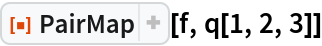Out=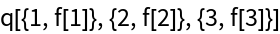A four-argument form of PairMap in which the function h is composed with the result:

 In:=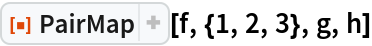Out=### Applications (3)

Use PairMap to create a Dataset in which the keys are integers and the columns are the squares and cubes of those integers:

 In:=Out=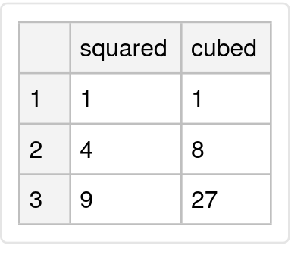Get data in a form suitable for statistical functions such as LinearModelFit:

 In:=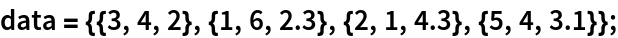In:=Out=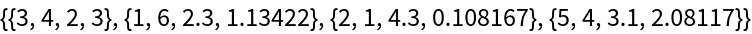Get data in a form suitable for machine learning functions such as Predict, Classify or NetTrain:

 In:=Out=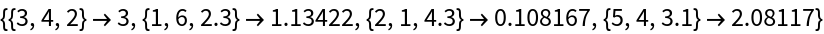### Properties and Relations (1)

Replicate the functionality of AssociationMap:

 In:=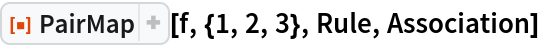Out=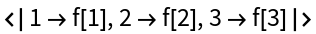### Neat Examples (1)

Take a complex model and build local emulators around a point {0.4,0.7} using LASSO with varying degrees of regularization in a process that approximates the LIME algorithm for explaining complex machine learning models:

 In:=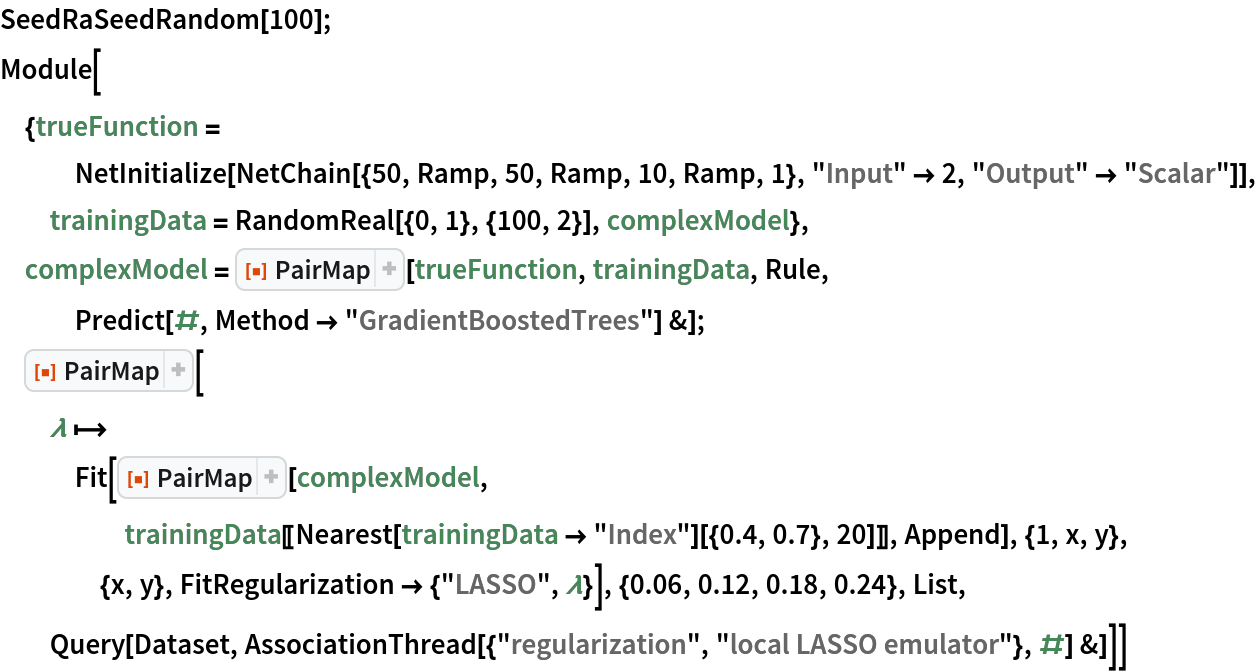Out=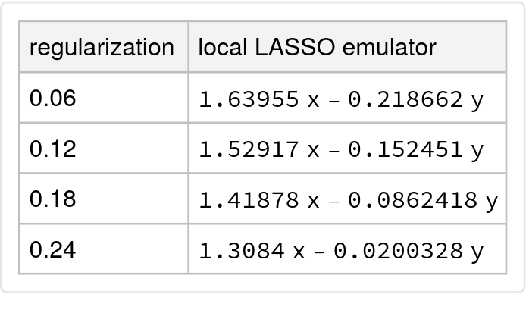Seth J. Chandler

## Version History

• 1.0.0 – 06 May 2020